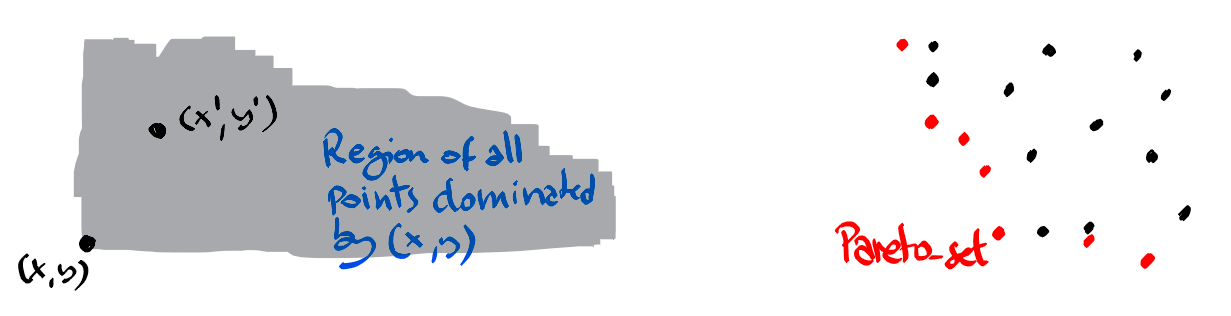Compute the pareto set of a given set of points in 2 or 3 dimensions.

## Definition

A point $(x,y)$ dominates all points $(x’,y’)$ with $x\leq x’, y\leq y’$ where at least one inequality is strict. Given a point set the tasks is to determine all non-dominated points. These points form the so-called Pareto-set.## In 2 dimensions

In 2 dimensions we can process the points from left to right, and for points with the same x-coordinate from bottom up. This order ensures that when we process a point $p$, it cannot be dominated by points processed later.

Two important observations. If $p$ is dominated by $p’$ and $p’$ by $q$, then $p$ is also dominated by $q$. We say that domination is transitive. The result is that in order to decide if a currently processed point $p$ is dominated, we only need to check domination with the Pareto set of the already processed points. And the second observation is that if $p$ is dominated by some point $q$ in the Pareto set then it is also dominated by the last point added to the current Pareto set.

As a result we only need to compare each point with the last added point from the Pareto set.

The overall complexity is $O(n\log n)$ which comes from the initial sorting.

## In 3 dimensions

Again we process the points in lexicographical increasing order. Every point is given by its coordinates $(x,y,z)$. For technical reasons we want to associate to $y$ its rank rank[y] among all the $y$-coordinates of the given points. This does not affect the domination order, and will allow us to use the rank as an index in a table $R$. It will be clear in a second, why this is useful.

To simplify the implementation, if there are multiple $y$-values among the given points, then we allow the ranks to be not successive. We only need them to be in order and bounded by $n$, the number of given points. For example if the $y$-values are $[1,4,4,4,6,7]$, then we will give the ranks rank=0, rank=3, rank=4, rank=5.

Again some observations. When processing point $p=(p_x,p_y,p_z)$, we only need to verify if it is dominated among the already processed points. And those already processed points have $x$-coordinate smaller equal $p_x$. So the question is if among those points there is one which has its $y$-coordinate at most $p_y$ and its $z$-coordinate at most $p_z$.

Here the table $R$ comes at hand. We store in $R[i]$ the smallest value $z$ such that we have seen a point $(x,y,z)$ with rank[y]==i. If there is no such point we set rank[y]=$+\infty$. Hence $p$ is dominated if and only if

$\min\{R, R,\ldots, R[i]\} \leq p_z$

for $i$ being the rank of $p_y$. A Fenwick or Segment tree can be used to store the table $R$ and allow to perform the above minimum query and update of R[i] in logarithmic time.

Small detail: if a point appears several times in the input, then its copies will be processed one after another. If it is a non-dominated point, then the second copy would appear as dominated by the above described test. But this can be handled correctly, by comparing each processed point, with the last point added to the Pareto set.

This leads to the following algorithm with an overall complexity of $O(n\log n)$.

## Fenwick Min Tree

The above code uses a variant of the Fenwick tree, which allows to store a table $R$, initially filled with $+\infty$, the neutral element for the min operator. Now we can either update $R$ at a given index $i$ and decrease its value to a given value $val$. If $R[i]$ is already smaller than $val$, then the update does nothing. And given an index $i$ we can query the minimum among $R,R,\ldots, R[i]$. All these operations can be performed in logarithmic time, in the size of the table.